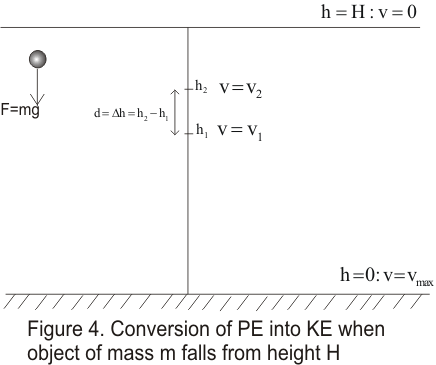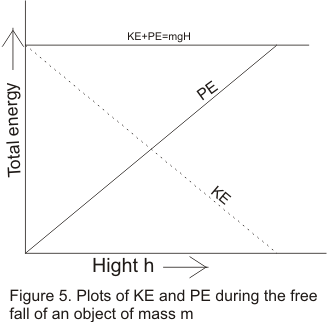# Potential energy|Gravitational Potential Energy

## (6)Potential energy

• Potential energy is the energy stored in the body or a system by virtue of its position in field of force or by its configuration
• Force acting on a body or system can change its Potential energy
• Few examples of bodies possesing Potential energy are given below
i) Stretched or compressed coiled spring
ii) Water stored up at a height in the Dam possess PE
iii) Any object placed above the height H from the surface of the earth posses PE
• Potential energy is denoted by letter U
• In next two topics we would discuss following two example of PE
i)PE of a body due to gravity above the surface of earth
ii) PE of the spring when it is compressed orr elongated by the application of some external force

## (7) Gravitational Potential energy(near the surface of earth)

• All bodies fall towards the earth with a constant acceleration known as acceleration due to gravity
• Consider a body of mass m placed at height h above the surface of the earth
• Now the body begins to fall towards the surface of the earth and at any time t ,it is at height h' (h' < h) above the surface of the earth
• During the fall of the body towards the earth a constant force F=mg acts on the body where direction of force is towards the earth
• Workdone by the constant force of gravity is
W=F.d
W=mg(h-h')
W=mgh-mgh' -(12)
From equation (12) ,we can clearly see that work done depends on tbe difference in height or position
• So a potential energy or more accurately gravitational potential energy can be associated with the body such that
U=mgh
Where h is the height of the body from the refrence point
• if
Ui=mgh Uf=mgh' or
W=Ui-Uf=-ΔU           (13)
The potential energy is greater at height h and smaller at lower height h
• We can choose any position of the object and fix it zero gravitational PE level.PE at height above this level would be mgh
• The position of zero Potential energy is choosen according to the convenience of the problem and generally earth surface is choosen as position of zero PE
• One important point to note is that equation (13) is valid even for the case when object object of mass m is thrown vertically upwards from height h to h'( h' > h)
• Points to keep in mind
i) If the body of mass m is thrown upwards a height h above the zero refrence level then its PE increase by an amount mgh
ii) If the body of mass falls vertically downwards through a height h ,the PE of body decreases by the amount mgh

## (8) Conversion of Gravitational Potential Energy to Kinetic Energy

• Consider a object of mass placed at height H above the surface of the earth
• By virtue of its position the object possess PE equal to mgH
• When this objects falls it begins to accelerate towards earth surface with acceleration equal to acceleration due to gravity g
• When object accelerates it gathers Speed and hence gains Kinetic energy at the expense of gravitational PE
• To Analyze this conversion of PE in KE consider the figure given below• Now at any given height h,PE of the object is given by the U=mgh and at this height speed of the object is given by v
• Change in KE between two height h1 and h2 would be equal to the workdone by the downward force
mv12/2 -mv22/2 =mgd           (14)
Where d is the distance between two heights .here one important thing to note is that both force and are in the same downwards direction
• Corresponding change in Potential energy
U1-U2=mg(h1-h2)=-mgd           (15)
Here the negative sign indicates the decrease in PE whch is exacty equal to the gain in KE
• From equation (14) and (15)
mv12/2 -mv22/2=U2-U1
or KE1 + PE1=KE2 + PE2
• Thus total energy of the system KE and gravitational PE is conserved .There is a conversion of one form of energy into another during the motion but sum remains same
• This can be proved as follows
At height H,velocity of the object is zero
ie
at H=H ,v=0 => KE=0
So $KE+PE=mgH$

at H=h ,v=v => KE=mv2/2=mg(H-h) and U=mgh
So KE+PE=mgH

at H=0 ,v=vmax => KE=mvmax2/2=mgH
So KE+PE=mgH
• This shows that total energy of the system is always conserved becuase total energy KE+PE=mgH ,which remains same as plotted in the figure given below• We know conclude that energy can neither be produced nor be destroyed .It can only be transformed from one form to another